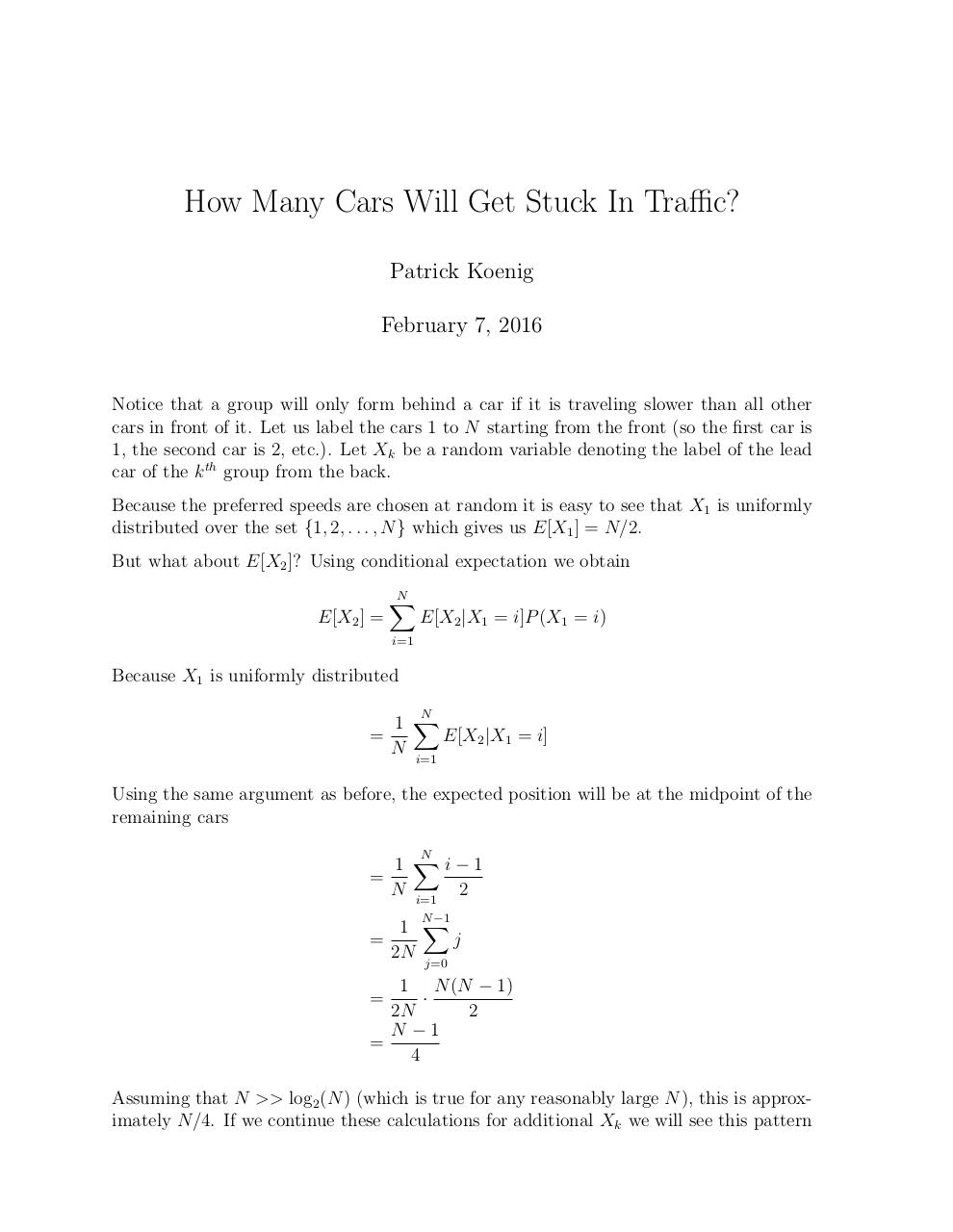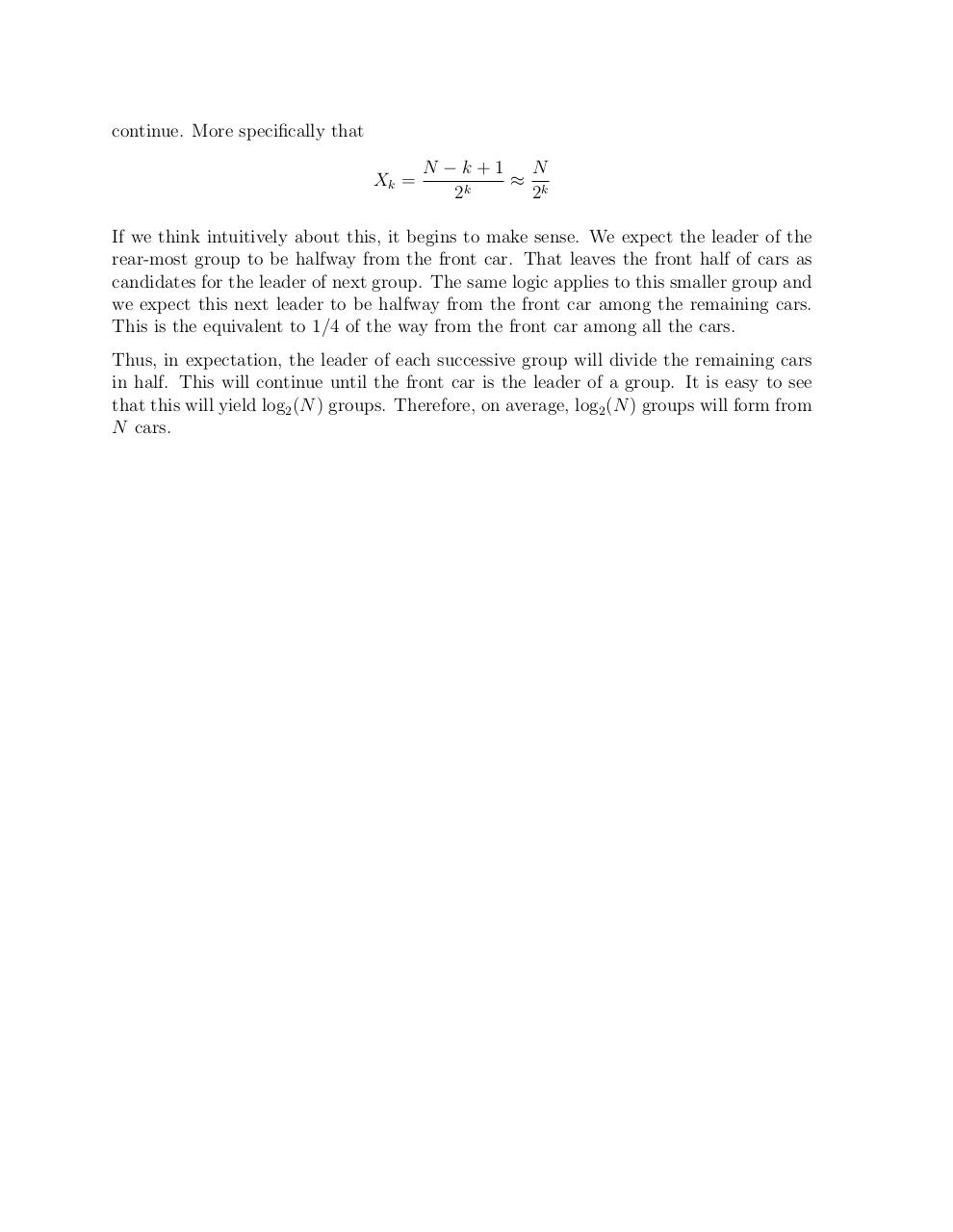# Riddler .pdf

### File information

Original filename: Riddler.pdf

This PDF 1.5 document has been generated by TeX / pdfTeX-1.40.16, and has been sent on pdf-archive.com on 07/02/2016 at 08:12, from IP address 73.154.x.x. The current document download page has been viewed 330 times.
File size: 80 KB (2 pages).
Privacy: public file

Riddler.pdf (PDF, 80 KB)

### Document preview

How Many Cars Will Get Stuck In Traffic?
Patrick Koenig
February 7, 2016

Notice that a group will only form behind a car if it is traveling slower than all other
cars in front of it. Let us label the cars 1 to N starting from the front (so the first car is
1, the second car is 2, etc.). Let Xk be a random variable denoting the label of the lead
car of the k th group from the back.
Because the preferred speeds are chosen at random it is easy to see that X1 is uniformly
distributed over the set {1, 2, . . . , N } which gives us E[X1 ] = N/2.
But what about E[X2 ]? Using conditional expectation we obtain
E[X2 ] =

N
X

E[X2 |X1 = i]P (X1 = i)

i=1

Because X1 is uniformly distributed
N
1 X
E[X2 |X1 = i]
=
N i=1

Using the same argument as before, the expected position will be at the midpoint of the
remaining cars
=

N
1 Xi−1
N i=1 2

=

N −1
1 X
j
2N j=0

1 N (N − 1)
·
2N
2
N −1
=
4

=

Assuming that N &gt;&gt; log2 (N ) (which is true for any reasonably large N ), this is approximately N/4. If we continue these calculations for additional Xk we will see this pattern

continue. More specifically that
Xk =

N −k+1
N

2k
2k

rear-most group to be halfway from the front car. That leaves the front half of cars as
candidates for the leader of next group. The same logic applies to this smaller group and
we expect this next leader to be halfway from the front car among the remaining cars.
This is the equivalent to 1/4 of the way from the front car among all the cars.
Thus, in expectation, the leader of each successive group will divide the remaining cars
in half. This will continue until the front car is the leader of a group. It is easy to see
that this will yield log2 (N ) groups. Therefore, on average, log2 (N ) groups will form from
N cars.#### HTML Code

Copy the following HTML code to share your document on a Website or Blog

#### QR Code### Related keywords